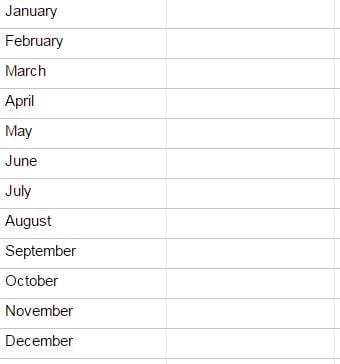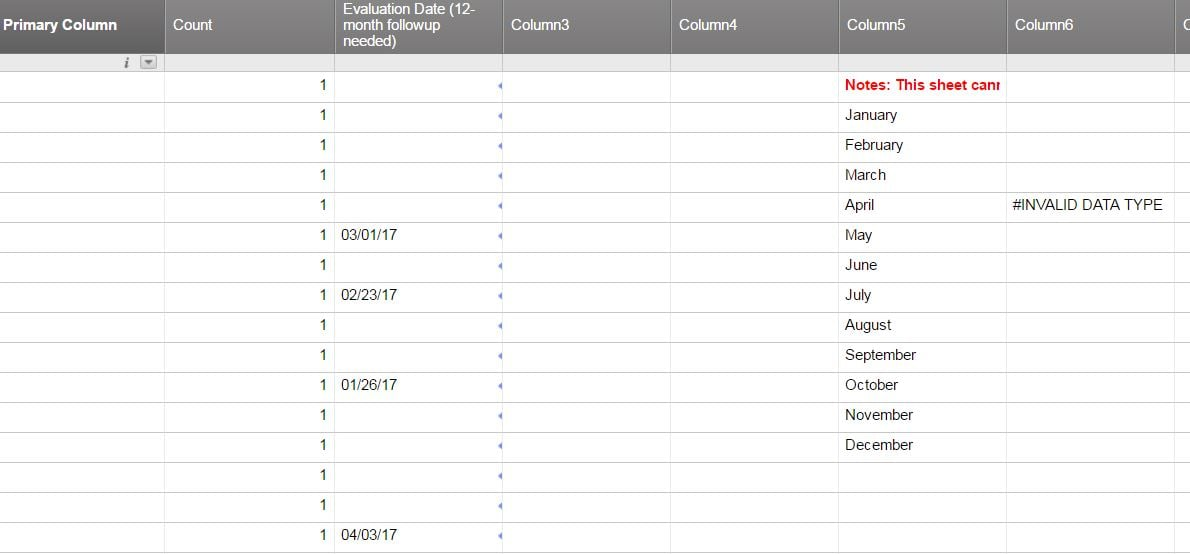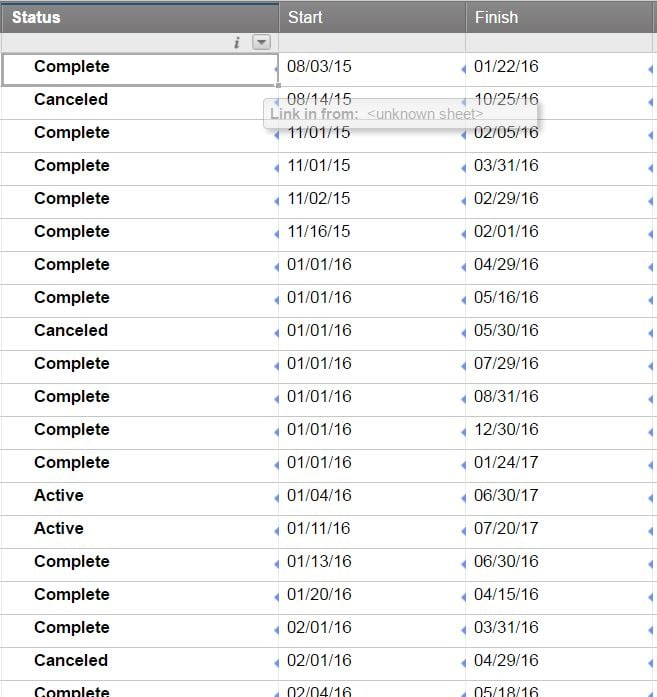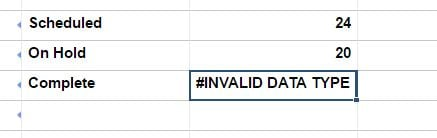#### Welcome to the Smartsheet Forum Archives

The posts in this forum are no longer monitored for accuracy and their content may no longer be current. If there's a discussion here that interests you and you'd like to find (or create) a more current version, please Visit the Current Forums.

# Using COUNTIF or SUMIF to evaluate a date range

Hi, so I have a ColumnA that has random dates for the current year 2017.  The column is formatted as a "Date" style column.

My goal is to sum each date in this ColumnA by Month:

Example:

So to the right of each date, I want the sum of all dates that are in "January" that are in Column A.

Thanks• I have some previous answers, so these are based on that. For an arbitrary date range, the formula looks something like this:

=SUMIF([Due Date]1:[Due Date]6, AND(@cell >= DATE(2017, 4, 1), @cell < DATE(2017, 5, 1)), Cost1:Cost6)

Note that DATE(2017,4,1) could be substituted for a reference to a start date cell.

Similarly for the end date.

For month by month like you actually asked, you can do something like this:

=SUMIF([Due Date]1:[Due Date]6, AND(MONTH(@cell) = 4, YEAR(@cell) = 2017), Cost1:Cost6)

You can see I check for MONTH(@cell) = 4, and that just means April of course. If you had this refer to a month number in another column, you could fill this down and get what you want.

• Thanks for the reply.  I am getting "Invalid Data Type::

My formula:

=SUMIF([Evaluation Date (12-month followup needed)]1:[Evaluation Date (12-month followup needed)]16, AND(MONTH(@cell) = 4, YEAR(@cell) = 2017), Count1:Count16)

I am assuming your "Cost1:Cost6" was a separate column that had numbers for sum?  I changed mine to "Count".

Also, the date column is a link from another sheet.  I am doing this to create a reference sheet for a Sights widget.• Yes, Cost was another numeric.

The formula doesn't seem to work if you have blanks in the list. I don't think the date functions like that.

I think we can wrap the condition in "IFERROR" and then return false if it isn't a date.

=SUMIF([Evaluation Date (12-month followup needed)]1:[Evaluation Date (12-month followup needed)]16, IFERROR(AND(MONTH(@cell) = 4, YEAR(@cell) = 2017), false), Count1:Count16)

• This worked perfectly.  Thank you!

• Hi, I have a new request.

I am looking for a formula so I can use a widget that displays total completed projects for just 2017 using the count or sum formula.  I am using a reference sheet for the widget and linking from another sheet to access data.

In the attached picture, I want to count just the Completed from the Status column that have a 2017 "Finish" column Date.  Basically, excluding any Finish date from prior years.

Thank you for any help.• Try this:

=COUNTIFS(Status1:Status8, "Complete", Finish1:Finish8, NOT(ISBLANK(@cell)))

It's better to post a new question so everybody can see it and have a chance to respond, as I might miss this!

• Thank you for the reply.  This works, except I need to just count Completes with a Finish column date of 2017.  That Finish column also has 2016 completes in it that I do not want counted.

Also, I will add any other questions to a new thread.  Thanks again for any help!

• change the NOT(ISBLANK(@cell)) to YEAR(@cell)=2017

• No luck:

My formula

=COUNTIFS(Status1:Status500, "Complete", Finish1:Finish500, YEAR(@cell) = 2017)• Just as previously, the formulas do not like blank dates.

Try this:

=COUNTIFS(Status1:Status500, "Complete", Finish1:Finish500, IFERROR(YEAR(@cell) = 2017, false))

Craig

• Thank you.  It works!

• Hi, your solution to my last issue works perfectly, but I have a new ask:

How do I get that formula to also scan a second column for the same criteria as well?  For example, the second column to also check is named "Revised Finish".

Current working formula:

=COUNTIFS(Status1:Status500, "Complete", Finish1:Finish500, IFERROR(YEAR(@cell) = 2017, false))

Thank you for any information!

• I am trying to do something similar but can't get this to work.

I want to check all dates for items created in the current month.

=SUMIF(Created:Created, AND(MONTH(@cell) = 3, YEAR(@cell) = 2018)

What am I doing wrong? And can I make this a formula that understand what month it is instead of having to update the month number each month?

I also tried:

=COUNTIFS(Created:Created, AND(MONTH(@cell) = 3, YEAR(@cell) = 2018))

This discussion has been closed.